• matlab是一款功能强大的通用工程数学软件。...然而十分可惜的是与之对应的ezsurf和ezmesh却对隐函数曲面F(x,y,z)=0的绘制无能为力。那么matlab究竟有没有用来绘制诸如F(x,y,z)=0的命令呢？答案毫无疑问是肯定的。事实上

matlab是一款功能强大的通用工程数学软件。利用matlab的作图功能可以轻而易举地绘制出各种复杂函数图形。利用ezplot甚至可以直接绘制隐函数曲线F(x,y)=0而无需将其写成y=f(x)的形式。然而十分可惜的是与之对应的ezsurf和ezmesh却对隐函数曲面F(x,y,z)=0的绘制无能为力。那么matlab究竟有没有用来绘制诸如F(x,y,z)=0的命令呢？答案毫无疑问是肯定的。事实上利用matlab的等值面函数isosurface就可以轻松搞定。关于isosurface的具体用法大家可以自己参看help。下面两个函数就是我利用isosurface编写的通用隐函数曲面绘制命令。

1.隐函数曲面网格图函数implicitmesh

function h=implicitmesh(f,xlimit,ylimit,zlimit,gd)
%implicitmesh(f,span,gd):画隐函数曲面f(x,y,z)=0的网格图,
%                          各坐标范围均限定在span=[lb,ub],
%                          网格数为gd，默认为25
%implicitmesh(f,xspan,yspan,zspan,gd):画隐函数曲面f(x,y,z)=0,
%                          各坐标范围分别限定在xspan,yspan,zspan
%h=implicitmesh(...)：画隐函数曲面并输出句柄
%例一：
%implicitmesh(inline('x.*y+z.^2'),[-5 5])%注意*\^一定要设成点运算
%例二：
%f=@(x,y,z)x.^2+y.^2+0*z-1;%注意如果f中不含某个变量一定要加上诸如0*y的项。
%implicitmesh(f,[-1 1],10)
%例三：
%f=@(x,y,z)(x.^2 + (9/4)*y.^2 + z.^2 - 1).^3 - x.^2.*z.^3 - (9/80)*y.^2.*z.^3;
%g=@(x,y,z)(sqrt(x.^2+y.^2)-2).^2+z.^2-.09;
%implicitmesh(f,[-1.5 1.5],[-.8 .8],[-1.5 1.5],50);
%hold on%可以添加图形
%h=implicitmesh(g,[-2.3,2.3]);
%colormap hsv;set(h,'facecolor','none');%可以设置各种效果
%axis off;axis equal;
if nargin==2
ylimit=xlimit;zlimit=xlimit;gd=25;
elseif nargin==3
gd=ylimit;ylimit=xlimit;zlimit=xlimit;
elseif nargin==4
gd=25;
elseif nargin==5
else
error('Error in input arguments')
end
x=linspace(xlimit(1),xlimit(2),gd);
y=linspace(ylimit(1),ylimit(2),gd);
z=linspace(zlimit(1),zlimit(2),gd); [x,y,z]=meshgrid(x,y,z);val=f(x,y,z);
[f,v]=isosurface(x,y,z,val,0);
if isempty(f)
warning('There is no graph in the range.');
p=[];
else
newplot;
p=patch('Faces',f,'Vertices',v,'CData',v(:,3),'facecolor','w','EdgeColor','flat');
isonormals(x,y,z,val,p);view(3);grid on
end
if nargout==0
else
h=p;
end

效果图：2.隐函数曲面表面图函数implicitsurf

function h=implicitsurf(f,xlimit,ylimit,zlimit,gd)
%implicitsurf(f,span,gd):画隐函数曲面f(x,y,z)=0的网格图,
%                          各坐标范围均限定在span=[lb,ub],
%                          网格数为gd，默认为25
%implicitsurf(f,xspan,yspan,zspan,gd):画隐函数曲面f(x,y,z)=0,
%                          各坐标范围分别限定在xspan,yspan,zspan
%h=implicitsurf(...)：画隐函数曲面并输出句柄
%例一：
%implicitsurf(inline('x.*y+z.^2'),[-5 5])%注意*\^一定要设成点运算
%例二：
%f=@(x,y,z)x.^2+y.^2+0*z-1;%注意如果f中不含某个变量一定要加上诸如0*y的项。
%implicitsurf(f,[-1 1],10)
%例三：
%f=@(x,y,z)(x.^2 + (9/4)*y.^2 + z.^2 - 1).^3 - x.^2.*z.^3 - (9/80)*y.^2.*z.^3;
%g=@(x,y,z)(sqrt(x.^2+y.^2)-2).^2+z.^2-.09;
%h=implicitsurf(f,[-1.5 1.5],[-.8 .8],[-1.5 1.5],50);
%set(h,'AmbientStrength',.5);%可以设置各种效果
%hold on%可以添加图形
%h=implicitsurf(g,[-2.3,2.3],[-2.3,2.3],[-.3,.3]);
%colormap hsv;set(h,'AmbientStrength',.8,'FaceAlpha',.5);%可以设置各种效果
%axis off;axis equal;shading interp;camlight;lighting gouraud;
if nargin==2
ylimit=xlimit;zlimit=xlimit;gd=25;
elseif nargin==3
gd=ylimit;ylimit=xlimit;zlimit=xlimit;
elseif nargin==4
gd=25;
elseif nargin==5
else
error('Error in input arguments')
end
x=linspace(xlimit(1),xlimit(2),gd);
y=linspace(ylimit(1),ylimit(2),gd);
z=linspace(zlimit(1),zlimit(2),gd); [x,y,z]=meshgrid(x,y,z);val=f(x,y,z);
[f,v]=isosurface(x,y,z,val,0);
if isempty(f)
warning('There is no graph in the range.');
p=[];
else
newplot;
p=patch('Faces',f,'Vertices',v,'CData',v(:,3),'facecolor','flat','EdgeColor','k');
isonormals(x,y,z,val,p);view(3);grid on
end
if nargout==0
else
h=p;
end

效果图：将以上函数保存为m文件，文件名和函数名相同。

然后放到matlab工作目录（如work文件夹）下，之后就可在matlab中调用了。

用法可以直接help implicitmesh或help implicitsurf

展开全文• 首先三维数据如下，第一行第一列代表横纵坐标，然后其它的值是z值 然后要将3个维度的值分别做成一个矩阵 X: Y: Z: 然后在Matlab的工作空间里生产变量： 这样就可以使用surf函数了 效果图： ...
首先说明这里用的是离散的数据

首先三维数据如下，第一行第一列代表横纵坐标，然后其它的值是z值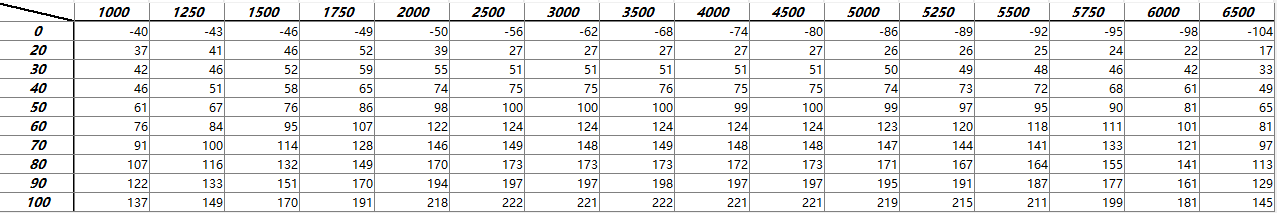然后要将3个维度的值分别做成一个矩阵

X: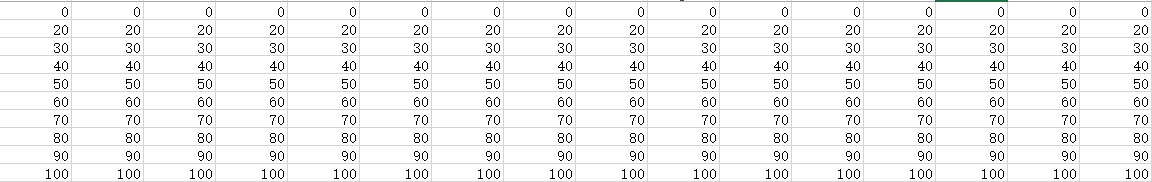Y: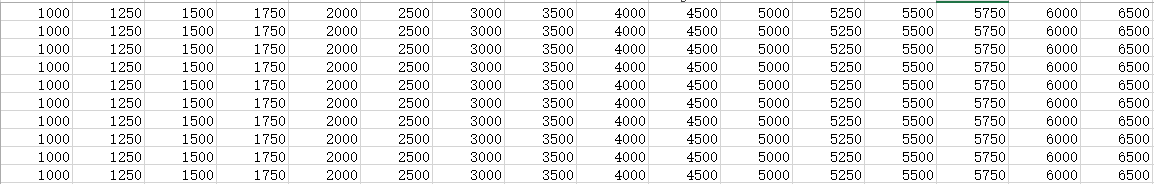Z: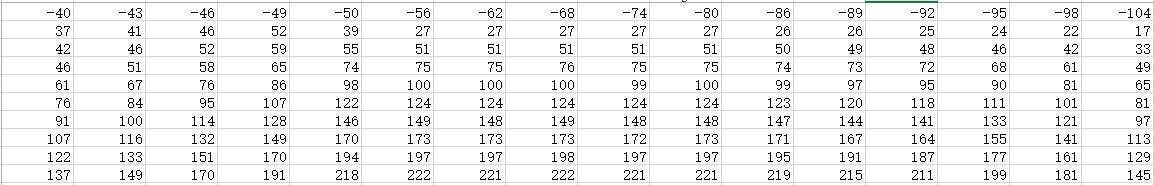然后在Matlab的工作空间里生产变量：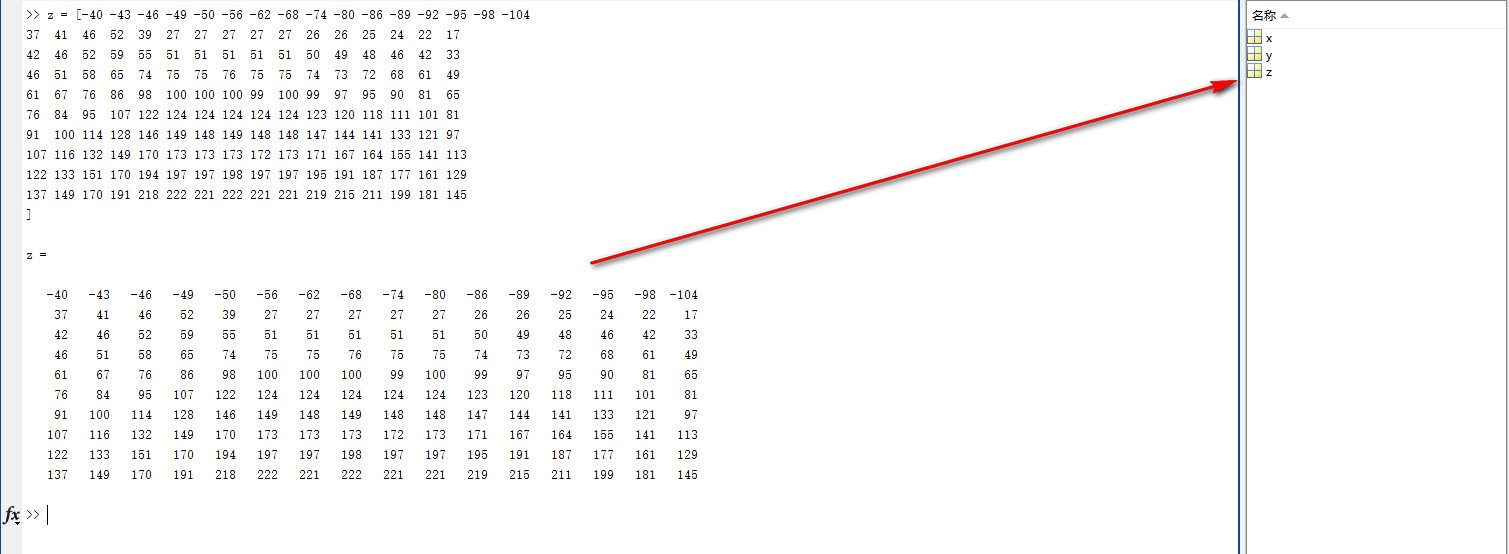这样就可以使用surf函数了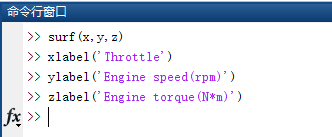效果图：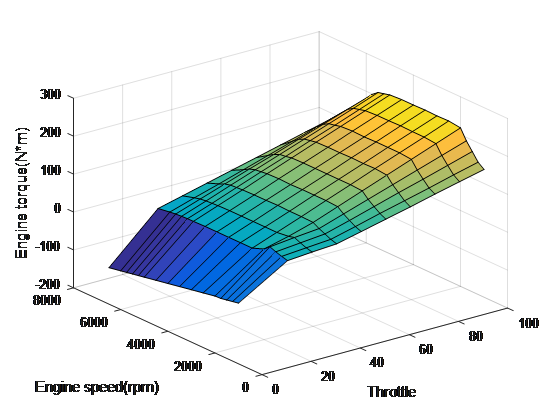展开全文• 画三维曲面之前，需要将三维曲面对应的平面坐标存储起来，怎么存储呢？很简单有两种方法 用矩阵X、Y分别存储每一个小矩形顶点的x坐标与y坐标，矩阵X、Y就是该矩形区域的xy平面网格坐标矩阵。 例如，在X-Y坐标平面...
文章目录0 前言1 平面网格数据的生成2 绘制三维曲面的函数2.1 mesh函数和surf函数的一般召唤方式2.2 其他召唤方式2.3 mesh、surf函数的亲戚朋友3.标准三维曲面3.1 sphere函数-画个球3.2 cylinder函数3.3 peaks 函数-峰值曲面图3.4 fmesh函数和fsurf函数-三个方程 两个自变量4 结语
0 前言
本文是科学计算与MATLAB语言的专题四的第5小节总结笔记，并结合了自己一点的理解，看完本文，可以轻松利用MATLAB相关函数，画出漂亮的三维曲面。
1 平面网格数据的生成
在画三维曲面之前，需要将三维曲面对应的平面坐标存储起来，怎么存储呢？很简单有两种方法。
用矩阵X、Y分别存储每一个小矩形顶点的x坐标与y坐标，矩阵X、Y就是该矩形区域的xy平面网格坐标矩阵。
例如，在X-Y坐标平面生成一个由(2,6),(3,8)围成的网格坐标。
在MATLAB中，产生平面区域内的网格坐标矩阵有两种方法。
利用矩阵运算生成
x=2:6;
y=(3:8)';
X=ones(size(y))*x;
Y=y*ones(size(x));

X和Y相同位置上的元素，如$X_{32}$、$Y_{32}$是区域的第3行第2列网格点的坐标（3，5）。
利用meshgrid函数生成
这种方法是我们需要的！简洁，明了！
[X，Y]=meshgrid（x，y）；
其中
参数x、y为向量，存储网格点坐标的X、Y为矩阵。
x=2:1:6；
y=(3:1:8)'；
[X,Y]=meshgrid(x,y)；

第3行命令生成的网格坐标矩阵X、Y与第一种得到的相同。
若根据每一个网格点上的x、y坐标求函数值z，则得到函数值矩阵Z。矩阵X、Y、Z中的各个列向量，对应于一条曲线数据点的坐标。
例1  绘制空间曲线。x = 2:6;
y = (3:8)';
[X, Y] = meshgrid(x, y);
Z = randn(size(X));
plot3(X,Y,Z)
grid on;

2 绘制三维曲面的函数
2.1 mesh函数和surf函数的一般召唤方式
mesh（x，y，z，c）
surf（x，y，z，c）
其中
x、y是网格坐标矩阵
z是网格点上的高度矩阵
c用于指定在不同高度下的曲面颜色
c省略时，颜色的设定正比于图形的高度。
例2  绘制三维曲面图$z=xe^{-x^2-y^2}$。t = -2:0.2:2;
[X, Y] = meshgrid(t);
Z = X .* exp(-X.^2 - Y.^2);
subplot(1,3,1)
mesh(X,Y,Z);
subplot(1,3,2)
surf(X,Y,Z);
subplot(1,3,3)
plot3(X,Y,Z);
grid on

2.2 其他召唤方式
mesh（z，c）
surf（z，c）
当x、y省略时，z矩阵的第2维下标当作x轴坐标，z矩阵的第1维下标当作y轴坐标。
例如，绘制一个曲面。t=1:5;
z=[0.5*t;2*t;3*t];
mesh(z);

注意：z是一个为3行5列的矩阵，因此X轴坐标为1、2 、3 、4、5，Y轴坐标为1、2、3.
2.3 mesh、surf函数的亲戚朋友

函数名称
函数说明

meshc
带等高线的三维网格曲面

meshz
带底座的三维网格曲面

surfc
带等高线的曲面

surfl
带光照效果的曲面

例3  用4种方式绘制函数$z=(x−1)^2+(y−2)^2−1$的曲面图。其中，$x∈[0,2]，y∈[1,3]。$[x,y]=meshgrid(0:0.1:2,1:0.1:3);
z=(x-1).^2+(y-2).^2-1;
subplot(2,2,1);
meshc(x,y,z);title('meshc(x,y,z)')
subplot(2,2,2);
meshz(x,y,z);title('meshz(x,y,z)')
subplot(2,2,3);
surfc(x,y,z);title('surfc(x,y,z)')
subplot(2,2,4);
surfl(x,y,z); title('surfl(x,y,z)')

3.标准三维曲面
3.1 sphere函数-画个球
[x，y，z]=sphere（n）
产生3个（n+1）阶的方阵，采用这3个矩阵可以绘制出圆心位于原点、半径为1的单位球体。
3.2 cylinder函数
[x，y，z]=cylinder（R，n）
其中，参数R是一个向量，存放柱面各个等间隔高度上的半径，n表示在圆柱圆周上有n个间隔点，默认有20个间隔点。
例4  用cylinder函数分别绘制柱面、花瓶和圆锥面。subplot(1,3,1);
[x,y,z]=cylinder;
surf(x,y,z);
subplot(1,3,2);
t=linspace(0,2*pi,40);
[x,y,z]= cylinder(2+cos(t),30);
surf(x,y,z);
subplot(1,3,3);
[x,y,z]= cylinder(0:0.2:2,30);
surf(x,y,z);

例5  用cylinder函数绘制两个相互垂直且直径相等的圆柱面的相交图形。[x,y,z]= cylinder(1,60);
z=[-1*z(2,:);z(2,:)];
surf(x,y,z)
hold on
surf(y,z,x)
axis equal

3.3 peaks 函数-峰值曲面图
peaks函数的召唤方式：
peaks（n）返回一个 n×n 矩阵
peaks（V）返回一个 n×n 矩阵，其中 n = length(V)
peaks（x，y）在给定的 X 和 Y（必须大小相同）处计算 peaks 并返回大小相同的矩阵
peaks
具体使用方式参考官方文档。
多峰函数:$z = 3(1-x)^2e^{-(x^2) - (y+1)^2 }-10(\frac{x}{5} - x^3 - y^5)e^{(-x^2-y^2})-\frac{1}{3}e^{-(x+1)^2 - y^2}$[x,y]=peaks(10);
z =  3*(1-x).^2.*exp(-(x.^2) - (y+1).^2) ...
- 10*(x/5 - x.^3 - y.^5).*exp(-x.^2-y.^2) ...
- 1/3*exp(-(x+1).^2 - y.^2) ;
surf(z)

也可以通过以下命令绘制出此图。
figure
peaks(10)是不是一样？好像不是，坐标轴间隔、标注不一样呀，哈哈哈。目前我也不知道这个函数有啥用，不过好像很厉害的亚子!
3.4 fmesh函数和fsurf函数-三个方程 两个自变量
如果一个图形由三个参数方程决定，有两个自变量，那么可以使用fmesh函数和fsurf函数。
fmesh(funx，funy，funz，uvlims）
fsurf(funx，funy，funz，uvlims）
其中，funx、funy、funz代表定义曲面x、y、z坐标的函数，通常采用函数句柄的形式。
uwlims为funx、funy和funz的自变量的取值范围，用4元向量（umin，umax，vmin，vmaxd描述，默认为[-5，5，-5，5]。
例6  绘制螺旋曲面\left\{ \begin{aligned} x&=usinv\\ y&=-ucosv,(-5 \leq u\leq5,-5\leq v\leq2)\\ z&=v \end{aligned}\right.
funx = @(u,v) u.*sin(v);
funy = @(u,v) -u.*cos(v);
funz = @(u,v) v;
fsurf(funx,funy,funz,[-5 5 -5 -2])%画出下半部分
hold on
fmesh(funx,funy,funz,[-5 5 -2 2])%画出上半部分
hold off

4 结语
您是否学会了呢？如果本文对您有帮助，可以点个赞哈，如有错误疑问，请您指出哈。


展开全文线性代数 算法 windows
• %画三维曲面 %Z为X,Y显函数 clc clear x=-8:0.2:8; y=x; [X,Y]=meshgrid(x,y); R=sqrt(X.^2+Y.^2); Z=sin(R)./R; surf(X,Y,Z) %画三维曲面 %Z不是X,Y显函数 clc clear x=-3:0.2:3; y=x; z=x; [X,Y,Z]=meshgrid(x,y,...
%画三维曲面
%Z为X,Y显函数
clc
clear
x=-8:0.2:8;
y=x;
[X,Y]=meshgrid(x,y);
R=sqrt(X.^2+Y.^2);
Z=sin(R)./R;
surf(X,Y,Z)

%画三维曲面
%Z不是X,Y显函数
clc
clear
x=-3:0.2:3;
y=x;
z=x;
[X,Y,Z]=meshgrid(x,y,z);
f=X.^2+Y.^2+Z.^2-1;
patch(isosurface(x,y,z,f,0))



展开全文• MATLAB绘制3D隐函数曲面的方法总结-MarchingCubes.zip 本帖最后由 winner245 于 2013-10-28 00:45 编辑 背景介绍 Matlab提供了一系列绘图函数，常见的包括绘制2D曲线的plot函数、绘制2D隐函数曲线的ezplot函数...
• 1.一个曲面使用函数 surf clc,clear, m=1:1:200; n=1:200; sigma = 200; f = fspecial('gaussian', [length(m),length(n)], sigma); %创建预定义的二滤波器 figure, surf(m,n,f); %m为横坐标，n为纵坐标。f点...
• matlab画三维图在Matlab中，三维图形的绘制包括三维曲线，三维网线图和三维曲面图。闲话不多说，直接进入正题。首先介绍几个函数：1.plot3(x,y,z,…)其中，x,y,z为维数相同的向量，分别储存3个坐标值；2.stem3(x,y,z...
• 用法 contour(z) 把矩阵z中的值作为一个二维函数的值，等高曲线是一个平面的曲线，平面的高度v是Matlab自动取的； contour(x,y,z) (x,y)是平面z=0上点的坐标矩阵，z为相应点的高度值矩阵。效果同上； contour...
• ＭＡＴＬＡＢ绘制三维图的程序　在MATLAB中，利用meshgrid函数产生平面区域内的网格坐标矩阵。mesh(x,y,z,c)：网格曲面，将数据点在空间中描出,并连成网格。 surf(x,y,z,c)：完整曲面，将数据点所表示曲面画出...
• 2 基本XYZ立体绘图命令mesh和plot是三度空间立体绘图的基本命令，mesh可出立体网状图，plot则可出立体曲面图，两者产生的图形都会依高度而有不同颜色。下列命令可出由函数形成的立体网状图: x=linspace(-2, 2,...
• 符号函数三维图形绘制1.plot3函数2.fplot3函数3.fmesh 三维网格图4.fsurf 三维曲面图5.fcontour 绘制等高线二.Matlab绘制隐函数的图形1. fimplicit3(f)2.fimplicit3(f,interval) 一.符号函数三维图形绘制 1.plot...数学建模 可视化 大数据
• ＭＡＴＬＡＢ绘制三维图的程序　在MATLAB中，利用meshgrid函数产生平面区域内的网格坐标矩阵。mesh(x,y,z,c)：网格曲面，将数据点在空间中描出,并连成网格。 surf(x,y,z,c)：完整曲面，将数据点所表示曲面画出。...
• matlab画三维图的方法（函数）可以分为如下类别： 1.三维曲线 代表函数：plot3 2.三维曲面 代表函数：surf、mesh、pcolor 注：绘图函数有很多，上面未完全列出。 绘制三维曲面时一定要注意数据的准备： ...
• 2 基本XYZ立体绘图命令mesh和plot是三度空间立体绘图的基本命令，mesh可出立体网状图，plot则可出立体曲面图，两者产生的图形都会依高度而有不同颜色。下列命令可出由函数形成的立体网状图: x=linspace(-2, 2,...
• 第二个子图：同时用plot函数绘制以红线、（2，3）为圆心、半径为2的圆，用函数ezplot绘制以（1，2）为圆心，半径为1.5的圆。标题为“圆”，图例为“plot”、“ezplot”，各自的圆心用句号“。”在对应位置标出。 ...
• 毕业季马上要到了，给大家总结一些我平常用到的奇特但是好用的Matlab画图技巧光滑变化的三维曲面三维曲面展示是目前MATLAB上人眼最容易直观观察的多维矩阵内容一种形式，因此三维矩阵的画图在很多的论文里都有涉及。...
• 三维曲面方程都可以写为 f(x,y,z)=0的方程形式 那么我们首先确定一个三维的空间网格x，y，z [x y z]=meshgrid(x1:dx:x2,y1:dy:y2,z1:dz:z2) 其中x1:dx:x2确定网格x方向的范围和间隔，y，z方向依次类推 当三者一样...
• 一、网格图形绘制以绘制函数z=f(x,y)三维网格图为例，下面为绘制步骤：确定自变量x和y的取值范围和取值间隔x = x1:dx:x2; y = y1:dy:y2;2.构成xoy平面上的自变量采样格点矩阵1) 利用“格点”矩阵生成原理生成矩阵X =...
• 2 基本XYZ立体绘图命令mesh和plot是三度空间立体绘图的基本命令，mesh可出立体网状图，plot则可出立体曲面图，两者产生的图形都会依高度而有不同颜色。下列命令可出由函数形成的立体网状图:x=linspace(-2, 2, ...
• Matlab自带很多有意思的彩蛋命令，包括二维图像、三维图像、动画、声音等等。在命令行窗口直接输入以下命令即可看到彩蛋。1、logomatlab的logo。2、membranematlab的logo的曲面。3、peaks峰值函数，本质是二元高斯...
• ezplot3 画三维曲线的简捷指令 ezpolar 画极坐标图的简捷指令 ezsurf 画表面图的简捷指令 ezsurfc 画带等位线的表面图的简捷指令 F f factor 符号计算的因式分解 feather 羽毛图 feedback 反馈连接 ...
• mesh和plot是三度空间立体绘图的基本命令，mesh可出立体网状图，plot则可出立体曲面图，两者产生的图形都会依高度而有不同颜色。下列命令可出由函数 形成的立体网状图: x=linspace(-2, 2, 25); % 在x轴上取...
• 1 目的 将4组三维数据，每组数据散点图（fig1），拟合出一个曲面（fig2），并将特定Z值的等高线投影到XY平面（fig3）。2 主要函数scatter3( ) 三维散点图scatter3(X,Y,Z,S,C) % 向量 X、Y 和 Z 指定点的位置，都...cell数组
• ## surf三维画图[matlab]

万次阅读 2017-10-21 10:27:43
如果想看用mesh画三维图，可以看这里 surf 三维阴影曲面画图，常用的函数格式 surf(X,Y,Z) X,Y,Z都是矩阵值，规模相等 surf(x,y,Z)x,y是n和m长度的向量，Z是m*n的矩阵(这个也很少用到，最多还是上面那些)代码1:x ...
• 当原始数据x,y都是一维的，但不知道z关于x、y的表达式，却有与x、y一一对应的一维数组z，这种情况要怎么画三维曲面图呢？ 答：插值。把x、y、z都扩充成二维的就能画出三维曲面了 插值里面比较好用的函数，当属 ...
• 实用标准文案精彩文档实验六MATLAB数据可视化一、实验目的掌握MATLAB二维、三维图形绘制，掌握图形属性的设置和图形修饰；掌握图像文件的读取和显示。二、实验内容(1)二维图形绘制。(2)三维曲线和三维曲面绘制。(3)...
• MATLAB不但擅长於矩阵相关的数值运算，也适合用在各种科学目视表示 ...plot是绘制一曲线的基本函数，但在使用此函数之前，我们需先定义曲 线上每一点的x及y座标。下例可出一条正弦曲线： close# matlab画三维函数曲面matlab 订阅